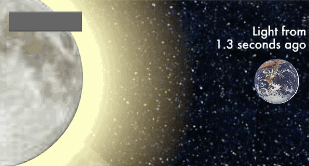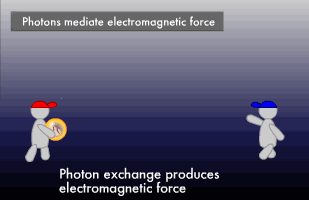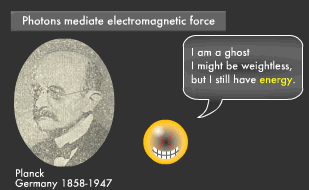# Canon Science Lab

## Light and Units

The speed of light is always constant. In the void of space, light travels at a constant speed, no matter what the inertial system. Let's look at light from the aspect of "unit."

The letter "c" represents the speed of light in formula:
c = 2.99792458 x 108 m/sec.
The speed of light will always be the same, no matter who measures it or how fast the measurer is moving. It is the fastest thing in the universe. Einstein is credited for this discovery.

### The Perpetually Constant Speed of Light

The speed of light is always constant. This is the foundation of the Special Theory of Relativity for uniform motion. (The General Theory of Relativity is a relative theory about accelerated motion). Based on this principle of the constancy of light velocity, the speed of light came to be used as the ultimate standard for length and time. The Special Theory of Relativity also led to the discovery that energy (E) and mass (m) are equivalent, which formulated E = mc2.The speed of light (c = 2.99792458×108 m/sec.) is equivalent to 299,792.458 km/sec. Light thus travels at nearly 300,000km/sec. Since the earth and moon are 380,000 km apart, light from the Moon takes about 1.3 seconds to reach us. And since the sun is 150 million km from the earth, the light we see right now left the sun about 8 minutes ago. A light year (the distance light travels in a year) is 9.5 trillion km.

### Are Units of Length Related to Light?

The basic unit of length is 1 m. The standard for units of length was once determined by the length of a person's stride or arm. Along with advances in science and technology came the need for a more accurate standard. In 1799, the French adopted a standard based on the size of the earth. They defined 1/10 millionth of the distance from the North Pole to the Equator as "1 meter."This is called the "standard meter." These days the standard meter is being redefined using the fact that light travels in a straight line at a constant speed. Accordingly, 1 meter is now defined as the distance light travels in 1/299.792458 million seconds. This definition allows us to use light to accurately measure the distance of remote objects. We can use a laser beam to measure the distance to the moon with extremely high accuracy (error of 30 cm or less). One second, the basic unit of time, is defined by the wavelength of a laser produced by cesium 133 atoms, but laser beams and light from space can also be employed for accurate time measurements.

### How Much Do Light Particles Weigh?

Once you know that light is the standard for measuring length and time, you might wonder whether light itself has weight. Light is both a wave and a particle. How much do you suppose such particles (photons) weigh? The answer is: photons have no mass. Photons are particles with zero mass, no electrical charge and a spin (rotation) value of 1. They can travel far because they weigh nothing. In quantum mechanics, photons are thought to mediate electromagnetic force.Although photons have no mass, there are extraordinary cases in which light, specifically evanescent light, clings to extremely small objects, behaving as if it had mass.

### Does Light Have Energy?

In 1900, Planck (a German physicist who lived from 1858 to 1947) announced oscillating electrons radiate electromagnetic waves with intermittent energy. Before that, it was thought that electromagnetic energy fluctuated continuously and could be endlessly split into smaller and smaller parts. According to Planck, energy is emitted in proportion to oscillation frequency.This proportionality constant is called "Planck's constant" (h = 6.6260755 x 10-34), and oscillation frequency times Planck's constant is known as an "energy quantum." If we try viewing this as light particles, we can consider electromagnetic waves of a certain oscillation frequency to be a group of photons with energy equal to oscillation frequency times Planck's constant. Photons are zero-mass particles, but because they have energy, they also possess momentum.